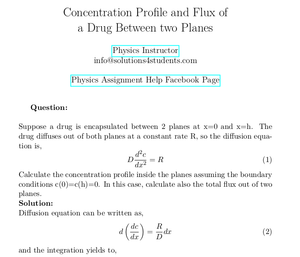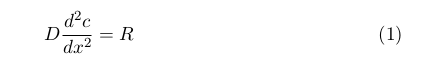Solved Problems in Physics, Physical Chemistry, and Computer Simulation Projects for College and Graduate Students.# Concentration Profile and Flux of a Drug Between two Planes

Regular price
\$6.50
Sale price
\$6.50
Tax included.

Question:
Suppose a drug is encapsulated between 2 planes at x=0 and x=h. The drug diffuses out of both planes at a constant rate R, so the diffusion equation is,Calculate the concentration profile inside the planes assuming the boundary conditions c(0)=c(h)=0. In this case, calculate also the total flux out of two planes.

By purchasing this product, you will get the step by step solution of the above problem in pdf format and the corresponding latex file where you can edit the solution. I am also available to help  you with any possible question you may have.